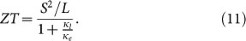## Introduction

Thermoelectric materials have attracted intense interest due to their potential applications in cooling and power generation1,2,3,4,5. The performance of thermoelectric devices depends on the dimensionless figure of merit, ZT, which is defined as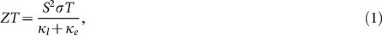where S is the Seebeck coefficient, T is the temperature, σ is the electrical conductivity, κl is the lattice thermal conductivity and κe is the thermal conductivity of the electronic carriers. To achieve a high ZT, it requires maximizing the Seebeck coefficient and electrical conductivity but minimizing the thermal conductivity. In general, the thermal conductivity can be minimized by introducing nanostructures into the thermoelectric materials to increase phonon scattering6,7,8, the Seebeck coefficient can be enhanced by quantum confinement effects9,10 and the electrical conductivity can be improved by adjusting the carrier concentration through doping11,12, however, the interdependence among these parameters makes the maximization of ZT a challenge so far.

Among various thermoelectric materials, Bi2Te3 and its solid solutions have been investigated intensely because they possess outstanding thermoelectric properties at room temperature, which is due to the high degeneracy at the edges of their energy bands. The ZT value of the Bi2Te3-based thermoelectric materials has been improved by many approaches such as the synthesis of nanostructured bulk materials or nanocomposites with a precise control of the size, structure, composition and carrier concentration as well as the deposition of various thin films by melt-spinning, sputtering, evaporation and molecular beam epitaxy13,14,15,16. Recently, Bi2Te3 has been demonstrated as a three-dimensional (3D) topological insulator, which further stimulates a strong research interest in this material17. Bulk Bi2Te3 crystallizes in a rhombohedral unit cell with the space group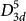(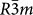) and has a layered structure with five atomic layers referred to as a quintuple layer (QL)18,19. The interaction between two adjacent QL is of the weak van der Waals type, which allows one to disassemble Bi2Te3 crystal into its quintuple building block20. The experimental results have shown that the exfoliated quintuples and ultrathin few-quintuple films have a low thermal conductivity, which is favorable to improve the thermoelectric performance20. Very recently, an efficient thermoelectric conversion was observed in the atomic monolayer steps of Bi2Te3 (n-type) and Sb2Te3 (p-type) QL by scanning photoinduced thermoelectric current probe21. Theoretically, P. Ghaemi et al. have discovered that nanometer-scale Bi2Te3 thin film generates a hybridization-induced band gap of the unconventional surface states, which can lead to an increased thermoelectric performance at low temperatures22. Recent theoretical researches show that Bi2Te3 nanofilms have enhanced thermoelectric properties18,23. B. Qiu et al. have studied the thermal conductivity of the few-quintuple Bi2Te3 nanofilms by using molecular dynamics simulation and have shown that nanoporous Bi2Te3 nanofilms display significantly reduced thermal conductivity as compared to the bulk couterparts24. All these results indicate that the few-quintuple Bi2Te3 nanofilms can be considered as promising thermoelectric materials.

In this work, we report an effective scheme of combining density functional theory and Boltzmann transport equations to provide a microscopic description of the thermoelectric properties of few-quintuple Bi2Te3 nanofilms. Our results demonstrate that p-type few-quintuple Bi2Te3 nanofilms can exhibit high thermoelectric performance and thus they can stand as a promising candidate for nanoscale thermoelectric applications.

## Computational methods

### First-principles calculation

The calculation of the energy band structure has been performed by using the projector augmented wave (PAW) method within the framework of density functional theory as implemented in the Vienna Ab initio Simulation Package (VASP)25,26,27. The exchange-correlation function is in the form of the Perdew-Burke-Ernzerh (PBE) with generalized gradient approximation (GGA)28,29. The Monkhorst-Pack scheme is adopted for the integration of Brillouin zone with a k mesh of 11 × 11 × 11 for the bulk Bi2Te3 and a k mesh of 11 × 11 × 1 for the Bi2Te3 few-quintuple films. In order to investigate the transport properties of the Bi2Te3 nanofilms, a denser k mesh of 45 × 45 × 1 is used. A cutoff energy of 450 eV is used in the plane wave basis. For the lattice constants one uses experimental value30 and interlayer separations for Bi2Te3 few-quintuple nanofilms are fully relaxed until the magnitude of the force acting on all atoms is less than 0.01 eV/Å and the total energy converges within 0.001 meV. The Bi2Te3 nanofilms with various thicknesses are modeled as a slab containing a few quintuples and a vacuum layer and Fig. 1 shows the models used in this simulation. For the five slabs with different thicknesses considered, a vacuum layer of 28 Å is added. Our tests revealed that this is sufficient for the prevention of spurious interactions between image slabs.

### Calculation of the transport properties

In this work, the semi-classical Boltzmann equations with the relaxation time approximation were used to evaluate the thermoelectric transport coefficients31. Previous calculations confirmed that it is an effective approach to predict the thermoelectric transport properties of bulk materials and nanosystems9,32,33. By solving the Boltzmann transport equations, σ, S and κe can be obtained as9,34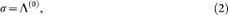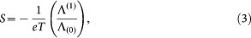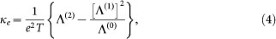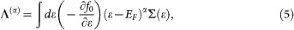where e is the charge of the carriers, T is the temperature, EF is the Fermi level, f0 is the Fermi-Dirac distribution function and ∑(ε) is the transport distribution function and can be expressed as31,35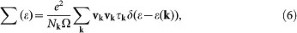where vk is the group velocity of the carriers associated with wave vector k, τk is the relaxation time, Nk is the number of the sampled k points in the Brillouin Zone (BZ) and Ω is the volume of unit cell. For a quasi-two-dimensional (2D) system, Ω should be substituted by the product of Γ and h, where Γ is the area of a 2D unit cell and h is the thicknesses of the quasi-2D system. As a result, for the quasi-2D system Eq. (6) can be expressed as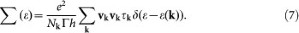Because the current version of the BoltzTrap program36 is restricted to analyze 3D materials, according to the discussion above we implemented the codes (modified from the BoltzTrap codes36) for quasi-2D systems to obtain the transport coefficients.

The relaxation time τ depends on the temperature, electronic energy and carrier concentration and generally is treated as a constant for convenience. Due to lack of available data for the relaxation time of Bi2Te3 nanofilms, we have adopted the bulk value as a reliable approximation by noting that the nanofilms possess similar covalent bonds with bulk Bi2Te323. Similar treatments have been done for nanoscale systems such as Si nanowires33, Bi2Te3 nanowires37, Bi2Te3 nanofilms23 and so on. For the relaxation time at 300 K, we used the value of 2.2 × 10−14 s, which T. J. Scheidemantel et al32 have adopted to study the thermoelectric properties of bulk Bi2Te3 and obtained consistent results with the experimental data38. The temperature dependence of the relaxation time of bulk Be2Te3 has been summarized in Table 137. The phonon thermal conductivity is another important parameter to determine the thermoelectric figure of merit. The previous theoretical study showed that phonon thermal conductivity of the perfect 1QL Bi2Te3 nanofilm decreases monotonically with temperature and exhibits a T−1 dependence23,24 and this inverse temperature dependence has also been found in bulk Bi2Te3 both theoretically39 and experimentally40. For the perfect Bi2Te3 nanofilms with a few QL, the inverse temperature dependence24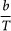will be adopted in our calculations and the coefficient b was determined by the value of the phonon thermal conductivity of Bi2Te3 nanofilms at 300 K24. Previous results showed that the thermal conductivity of few-quintuple Bi2Te3 nanofilms could be significantly reduced by introducing structure defects such as nanopore24. In the following, we also investigated the thermoelectric figure of merit of nanoporous Bi2Te3 nanofilms with few quintuples by assuming the defects don't significantly influence the electronic structure. Since the molecular dynamics simulation showed the thermal conductivity of nanoporous nanofilms depends weakly on the temperature and film thickness, we adopted a constant value of 0.3 W/mK in our calculation24.

## Results and discussion

### Band structure

The ab initio electronic band structures of Bi2Te3 bulk material and nanofilms with various thicknesses are shown in Fig. 2. The spin-orbit interaction was taken into account in the calculation, which was proved to be essential for calculating the electronic structures of Bi2Te3 and our results for bulk Bi2Te3 (Fig. 2(a)) are in good agreement with the data reported in previous work41. As shown in Figs. 2(b–f), band structures of the Bi2Te3 nanofilms are similar to each other except for the significant difference in band gap and energy spacing between adjacent bands. Firstly, the indirect band gap decreases with increasing the film thickness. For example, the band gap is about 320 meV for 1QL film (Fig. 2(b)) and it disappears when the film thickness increases to 3QL (Fig. 2(d)). Secondly, the energy spacing between the adjacent bands decreases with increasing the number of QL. The reasons are that with the thickness increasing, the coupling between the top and bottom electronic states and the quantum confinement effect on the electrons in the nanofilm are weakened. It is also noteworthy to point out that the conduction bands of all five nanofilms are much more dispersive than the valence bands.

The density of states (DOS) for the Bi2Te3 nanofilms with various thicknesses is shown in Fig. 3. The DOS near the edge of the valence band has a sharper and larger peak than that near the edge of the conduction band due to flatter valence bands (as shown in Fig. 2). It can be observed from Fig. 3 that the total DOS for 1 QL Bi2Te3 nanofilm displays staircase-like behavior near the edges of conduction bands and valence bands, induced by the quantum confinement effect in quasi-2D systems. With the number of QL decreasing, the spacing between the peaks (singularity) of the DOS significantly increases, which is consistent with the change of the energy spacing between the adjacent bands.

### Transport properties

Based on the calculated electronic band structures, the thermoelectric transport coefficients of the few-quintuple Bi2Te3 nanofilms can be evaluated by the methods presented in Sec. II. It is well known that the standard density functional theory usually underestimates the band gaps of semiconductors. In order to achieve a better agreement with the experimental results, the band gaps of the nanofilms were adjusted to match the experimental data42 by applying the so-called scissor operator in our calculations. Similar treatments have been widely adopted in previous publications43,44.

Fig. 4 shows the calculated electrical conductivity, Seebeck coefficient, power factor and figure-of-merit of the perfect p-type Bi2Te3 films with different QL and p-type bulk Bi2Te3 as a function of carrier concentration at 300 K. As shown in Fig. 4(a), the electrical conductivity of all the p-type Bi2Te3 nanofilms increases with increasing carrier concentration. In contrast, the Seebeck coefficient decreases with increasing carrier concentration and in general the Seebeck coefficient for the thinner nanofilm is larger than that for the thicker nanofilm at the same carrier concentration (Fig. 4(b)). This can be explained by the following discussion. Taking into account that Maxwell-Boltzmann approximation is justified in the region of EFEV kBT, S can be expressed as a function of n: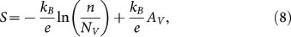where NV is the effective density of states at the edge of the highest valence band and AV represents a scattering factor of the semiconductor8. NV can be expressed as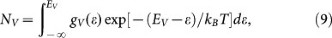where gV(ε) is the density of states. Eq. (8) directly reveals that the magnitude of S decreases with increasing n, as shown in Fig. 4(b). In addition, with the number of QL decreasing, the DOS near the edge of the valence band increases due to the quantum confinement effect (Fig. 3), leading to an increase of NV and hence an enhancement of S. It is worth noting that S of 1QL Bi2Te3 nanofilm is significantly larger than those of other nanofilms, which results from the significantly enhanced DOS near the edge of the valence band induced by the strong coupling between the top and bottom electronic states and the quantum confinement effect. The electrical conductivity σ increases with increasing carrier concentration n, whereas the Seebeck coefficient S decreases, therefore, there is an optimized carrier concentration, nopt, at which the power factor is maximized (as shown in Fig. 4(c)). The nopt to obtain the maximum power factor for Bi2Te3 nanofilms with various QL occurs in the range from 0.3 × 1020 cm−3 to 5 × 1020 cm−3. In general, the nopt increases with decreasing the number of QL. The nopt can be derived as8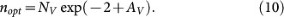Eq. (10) reveals that the nopt for the power factor is proportional to NV. With the number of QL decreasing, the DOS near the edge of the valence band increases, thus, NV rises and hence nopt increases (as shown in Fig. 4(c)). Figure 4(d) shows that the dependence of ZT on the carrier concentration is similar to that of the power factor, indicating that the carrier concentration is a key parameter to adjust the thermoelectric performance of the few-quintuple films. The nopt to obtain the highest ZT value is in the range from 0.1 × 1020 cm−3 to 1.5 × 1020 cm−3. The perfect 1QL film has the largest ZT value of 1.0 at an nopt of 1.5 × 1020 cm−3. The nopt for the ZT is different from that for the power factor because of the effect of the electronic thermal conductivity that is related to the carrier concentration. We have calculated the thermoelectric transport properties of bulk Bi2Te3 by using the value of 2.2 × 10−14 s at 300 K32 for the relaxation time and the value of 1.5 W/mK at 300 K32 for the phonon thermal conductivity. Our calculated results are in excellent agreement with the previous theoretical32 and experimental data38. It can be seen from Fig. 4 that the electrical conductivity of bulk Bi2Te3 is larger while the seebeck coefficient is smaller in most range of carrier concentrations, as compared with those of Bi2Te3 nanofilms at a fixed carrier concentration. The optimized ZT value of bulk Bi2Te3 is about 0.6, which is smaller than that of 1QL Bi2Te3 nanofilm (Fig. 4(d)).

Fig. 5 shows the calculated electrical conductivity, Seebeck coefficient, power factor and figure of merit of the p-type perfect 1QL Bi2Te3 nanofilm as a function of carrier concentration at different temperatures. As shown in Fig. 5(a), at a fixed carrier concentration the electrical conductivity decreases with increasing temperature, mainly because the scattering rate of the carriers increases and the relaxation time decreases with increasing temperature (as shown in Table 1). In contrast, the S slightly increases with increasing the temperature at a fixed carrier concentration, as shown in Fig. 5(b). This is mainly because when the temperature rises, the term, exp[−(EV − ε)/kBT] with ε < EV, in Eq. (9) increases and then it leads to an increasing NV. Due to the reduction of σ, the power factor decreases with increasing temperature and the nopt to obtain the maximum power factor shifts to a larger value (Fig. 5(c)). Fig. 5(d) shows that the dependence of ZT on the temperature is reversed as compared with that of the power factor. Although the power factor decreases with raising temperature, ZT value increases because both phonon and electron thermal conductivity decrease. The peak values of ZT for the p-type perfect 1QL Bi2Te3 film are 1.0 and 1.9 at 300 K and 800 K, respectively.